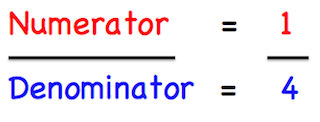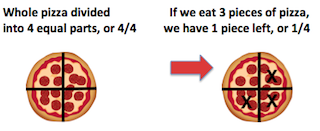# Numerator & Denominator Lesson for Kids

Instructor: Trisha Fyfe

Trisha has taught college and K-12 English, reading, writing, and math. She has a master's degree in teaching.

When we divide a whole into parts, we use fractions. There are all kinds of fractions: 1/2, 2/3, 3/4, and they all have something in common, a number above the line and another below. Let's learn about fractions and their numerators and denominators now.

## What Are Numerators and Denominators?

In math, there are times we have to divide something whole into pieces. Say, for instance, your family has one whole pizza to eat for dinner. If the pizza isn't cut into pieces, does that mean only one person gets to eat the whole pizza? Of course not! We would need to cut the pizza into equal pieces.

Let's say your dad cuts the pizza into 4 equal pieces. Because we have 4 equal pieces in our whole pizza, now your pizza becomes a fraction with a denominator of 4. The denominator shows us how many equal pieces something has been divided into. It is the bottom number in a fraction.

But wait! A fraction has a top number and a bottom number. If the bottom number is the denominator, that means the top number must have a name also, right? The top number in a fraction is the numerator. The numerator shows us how many pieces we are counting or working with.### Pizza Fractions

Back to the pizza! If your family eats 3 pieces of the pizza, how many pieces are left? There would only be 1 piece (out of 4) left. This means 1/4 of the pizza is left.Since we are counting only the leftover piece, this is numerator, and it goes on the top of our fraction. There were 4 equal pieces in our whole pizza, which means our denominator is 4. Remember the denominator is the number of equal pieces in our whole pizza.

### Puppy Fractions

But, it's not just pizza that we can turn into fractions. We can turn almost anything into parts and wholes. Here's another example: a group of 8 puppies, some are girls and some are boys. Let's make some fractions.

If there are 5 boy puppies and 3 girl puppies, how can we use fractions with numerators and denominators to represent these numbers?

To unlock this lesson you must be a Study.com Member.

### Register to view this lesson

Are you a student or a teacher?

#### See for yourself why 30 million people use Study.com

##### Become a Study.com member and start learning now.
Back
What teachers are saying about Study.com

### Earning College Credit

Did you know… We have over 200 college courses that prepare you to earn credit by exam that is accepted by over 1,500 colleges and universities. You can test out of the first two years of college and save thousands off your degree. Anyone can earn credit-by-exam regardless of age or education level.